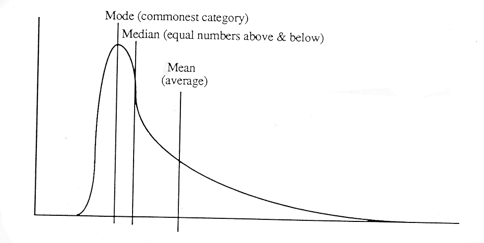# Other Distributions

Normally distributed data can be described by the mean and standard deviation (or variance). However, not normally distributed data requires further descriptive statistics. To describe the central tendency, the mode (most common category) and median (equal number of measurements above and below) are used in addition to the mean. The dispersion of data can be described with the range (interval from lowest to highest value), interquartile range (the difference between the upper and lower quartiles), or minimum and maximum values.

Distributions that are not Normal, are not necessarily symmetrical around the mean and can be skewed. The plot below shows the mode, median and mean in a right skewed distribution (a right skewed distribution has a ‘tail’ to the right, a left skewed distribution a ‘tail’ to the left):In a Normal distribution, the mean, mode and median are the same.